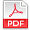## Scientific Review

Online ISSN: 2412-2599
Print ISSN: 2413-8835

# Archives

### Volume 4 Number 6 June 2018

#### The Formal-Logical Analysis of the Foundation of Set TheoryPDF

Authors: Temur Z. Kalanov
Pages: 53-63
Abstract
The critical analysis of the foundation of set theory is proposed. The unity of formal logic and rational dialectics is the correct methodological basis of the analysis. The analysis leads to the following results: (1) the mathematical concept of set should be analyzed on the basis of the formal-logical clauses “Definition of concept”, “Logical class”, “Division of concept”, “Basis of division”, “Rules of division”; (2) the standard mathematical theory of sets is an erroneous theory because it does not contain definition of the concept “element (object) of set”; (3) the concept of empty set (class) is a meaningless, erroneous, and inadmissible one because the definition of the concept “empty set (class)” contradicts to the definition of the logical class. (If the set (class) does not contain a single element (object), then there is no feature (sign) of the element (object). This implies that the concept of empty set (class) has no content and volume (scope). Therefore, this concept is inadmissible one); (4) the standard mathematical operations of union, intersection and difference of sets (classes) are meaningless, erroneous and inadmissible operations because they do not satisfy the following formal-logical condition: every separate element (object) of the set (class) must be in only one some set (class) and cannot be in two sets (classes). Thus, the results of formal-logical analysis prove that the standard mathematical theory of sets is an erroneous theory because it does not satisfy the criterion of truth.